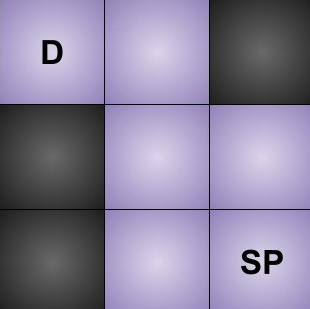# The Maze

Posted: 16 Mar, 2021
Difficulty: Moderate

## PROBLEM STATEMENT

#### The coordinates of starting point and destination are represented by row and column numbers.

##### For Example
``````Given maze: {[0, 0, 1], [1, 0, 0], [1, 0, 0]}
Starting point: [2, 2]
Destination: [0, 0]

For the above example maze will look like this:
````````````So, we can see there are 2 ways for Ninja to reach destination(D), from the starting point(SP). They are: [left -> up -> left] and [up -> left -> up -> left].
So, you need to print true.
``````
##### Input Format:
``````The first line contains an integer ‘T’ which denotes the number of test cases.

The first line of each test case contains two space-separated integers ‘M’ and ‘N’ denoting the dimensions of the maze.

The next ‘M’ lines contain ‘N’ space-separated integers each denoting the maze.

The next line of each test case contains two space-separated integers ‘startX’ and ‘startY’ denoting the coordinates of the starting point.

The last line of each test case contains two space-separated integers ‘destX’ and ‘destY’ denoting the coordinates of the destination.
``````
##### Output Format
``````For each test case, print a single line containing a single string containing ‘True’ if it is possible to reach the destination from the starting point and ‘False’ if it is not possible.

The output of each test case will be printed in a separate line.
``````
##### Note:
``````You do not need to print anything. It has already been taken care of. Just implement the given function.
``````
##### Constraints
``````1 <=T <= 50
1 <= M,N <= 100
0 <= MAZE[ i ] [ j ] <= 1

Where MAZE[ i ][ j ] is the value of the maze. The starting point and destination will always lie in the maze and will be in empty spaces.

Time limit: 1 sec.
``````Approach 1
• Ninja can move in at most four directions i.e left, right, up, and down.
• We will take a 2-D array ‘directions’ of size 4 x 2  in which the first column denotes the shift in the horizontal direction and the second column denotes a shift in the vertical direction.

We will store all the four directions { (0,1) (0,-1) ( 1,0) ( -1,0 ) )} in 2-d array.

• In each direction, Ninja continues to move until it will not reach the wall. For example - if Ninja is moving in the left direction, then he will continuously move in the left direction and will stop when reach the wall.
• Now we will check the stopping point in each direction. We will add the shift in horizontal and vertical direction until reach the wall. For example, if Ninja is moving in the right direction, we will continuously add (0,1) to the current coordinates of Ninja’s position.
• From the stopping point, he can again move in all four directions. So we will recursively solve for all directions from this new point.
• If any of the stopping points became equal to our destination, then we can say that we can reach the destination.

There will be many cases in which we will stop at a cell that is already explored. In order to not explore that cell again, we will maintain an extra array ‘visited’.

Algorithm

• If coordinates of start = coordinates of destination then return true.
• If the current cell is already visited i.e visited[startX][startY] = true, return false.
• Otherwise mark current cell as visited.
• Initialize a 2-D matrix ‘directions’ with values [ [ 0 1 ] , [ 0 -1 ] [ 1 0 ] [ -1 0 ] ].
• Let startX and startY be the x and y coordinates of starting position and destX and destY be the coordinates of the destination.
• Let shiftX and shiftY be variables that store shift in x and y-direction.
• Run a loop i: 0 to 3  for all the four directions
• Let currentX and currentY be two variables and store startX and startY to it.
• shiftX = directions [ i ][ 0 ] and shiftY = directions[ i ][ 1 ].
• Run a loop while currentX + shiftX is between 0 and length of the maze and currentY + shiftY is between 0 and length of the maze and also maze [ currentX ] [ currentY ] is not equal to ‘1’.